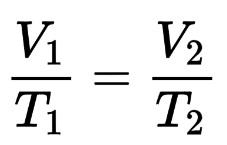# Charles Law Calculator

To use Charles law calculator, fill the required input boxes and click the calculate button

Formula:
T2 = V2 x T1 V1
L
K
L

Give Us Feedback

## Charles Law Calculator

Charles law calculator is used to find the initial & final volume and the initial & final temperature of an ideal gas. This tool calculates the missing values of the formula of the Charles law.

## What is Charles's law?

Charles law is a statement that the volume occupied by a fixed amount of gas is directly proportional to its absolute temperature if the pressure remains constant.

## Formula of Charles law## How to calculate the volume of gas using Charles's law?

Below is a solved example of Charles's law.

Example

Find the final volume (V2) of the gas by using Charles law, if V1 = 12L, T1 = 4K, & T2 = 8k.

Solution

Step 1: Identify the given terms of volume and temperature.

Initial temperature = T­1 = 4k

Final temperature = T2 = 8k

Initial volume = V1 = 12L

Final volume = V2 =?

Step 2: Substitute the given terms in the formula of Charles's law.

V1/T1 = V2/T2

12L/4K = V2/8k

(12L/4K) * 8k = V2

3L * 8 = V2

24L = V2

Hence the final volume of the gas is 24L.

### Math Tools